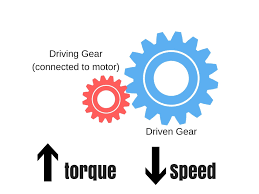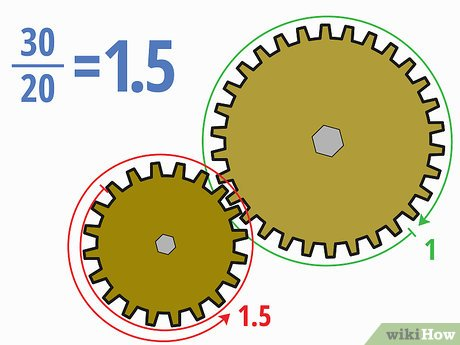# How to calculate the output torque of motor in ebike wheel ?

Torque and output power

The Brushless electric motor Calculate formula: Power (KW) = Torque (Nm) X RPM /9549So under the condition of constant power, the slower the speed, the greater the torque

How to calculate the wheel output power ?
Caluclate formula:Input torque * transmission ratio = output torque

For example:
FLIPSKY 6354 140 KV controlled by a VESC 4.20 Aluminium Heat sink on a bike with a 36 Volts battery (10S4P). The ratio of the gear is 22:248 The motor torque should be around 5Nm, and I expect minimum 50Nm wheel torque.

Question:

minimum 50Nm wheel torque can be achieved ?Calculate according to the above formula:
Input torque * transmission ratio = output torque
5Nm * (248:22)=56.36Nm

So the minumum 50Nm can be achieved.